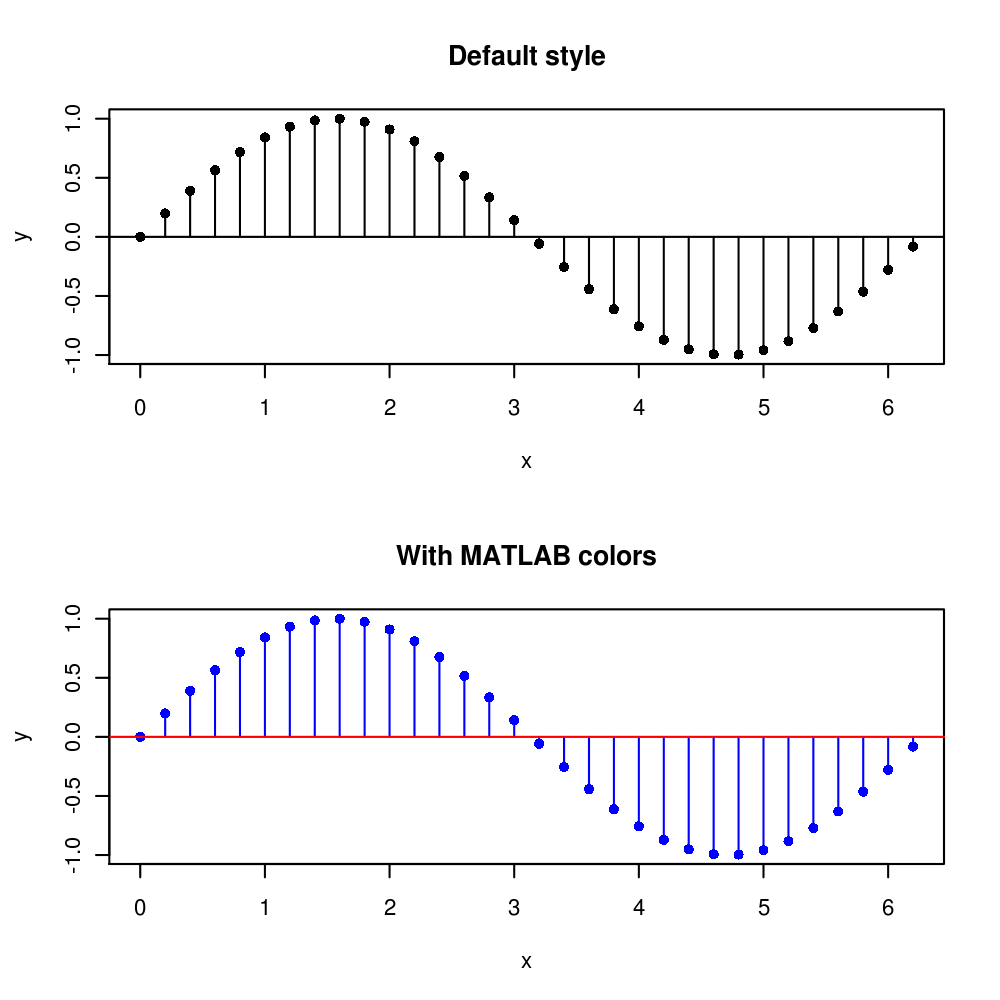Want to share your content on R-bloggers? click here if you have a blog, or here if you don't.

Recently I wanted to plot an impulse response function with R and missed the MATLAB style stem plot for doing it. I couldn’t find an R function for it with a quick Google search so I made my own. So here is the function and a small example :

```#The function
stem <- function(x,y,pch=16,linecol=1,clinecol=1,...){
if (missing(y)){
y = x
x = 1:length(x) }
plot(x,y,pch=pch,...)
for (i in 1:length(x)){
lines(c(x[i],x[i]), c(0,y[i]),col=linecol)
}
lines(c(x-2,x[length(x)]+2), c(0,0),col=clinecol)
}

#An example
x <- seq(0, 2*pi, by = 0.2)
par(mfrow=c(2,1))
stem(x,sin(x), main = 'Default style')
stem(x,sin(x),main='With MATLAB colors', col=4,linecol=4,clinecol=2)
```